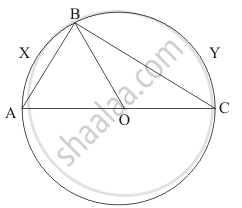Advertisement Remove all ads

# In the given figure, if O is the circumcentre of ∠ABC, then find the value of ∠OBC + ∠BAC. - Mathematics

Answer in Brief

In the given figure, if O is the circumcentre of ∠ABC, then find the value of ∠OBC + ∠BAC.Advertisement Remove all ads

#### SolutionSince, O is the circumcentre of   $\bigtriangleup ABC$, So, O would be centre of the circle passing through points AB and C

$\angle ABC$= 90°             (Angle in the semicircle is 90°.)
$\Rightarrow \angle OAB + \angle OBC = 90°$            .....(1)

As OA = OB    (Radii of the same circle)

$\therefore \angle OAB = \angle OBA \left( \text{ Angle opposite to equal sides are equal } \right)$

$\text{ or } , \angle BAC = \angle OBA$

$\text{ From } \left( 1 \right)$

$\angle BAC + \angle OBC = 90°$

Is there an error in this question or solution?
Advertisement Remove all ads

#### APPEARS IN

RD Sharma Mathematics for Class 9
Chapter 15 Circles
Q 8 | Page 109
Advertisement Remove all ads

#### Video TutorialsVIEW ALL 

Advertisement Remove all ads
Share
Notifications

View all notifications

Forgot password?
Course